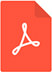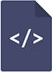# An idea about simple derivation of mean activity, mean activity coefficient, and mean molar concentrationPDF Full Text

# Abstract

A simple derivation procedure of equations about a mean activity, a mean activity coefficient, and a mean molar concentration was proposed based on the electrochemical potentials. The equations for the electrolytes, such as KCl, CaCl2 , and LaCl3 , were derived.

# Keywords

mean activity, mean activity coefficient, mean molar concentration, electrochemical potentials, strong electrolytes, average potential